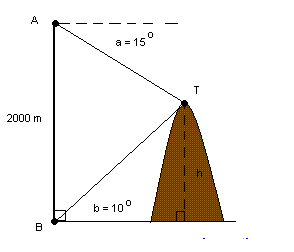# Trigonometry Practise

Geometry Level 3When the top T of a mountain is viewed from point A, 2000 m from ground, the angle of depression a is equal to 15 degrees and when it is viewed from point B on the ground the angle of elevation b is equal to 10 degrees . If points A and B are on the same vertical line, find the height h of the mountain. (round answer to one decimal place).

×